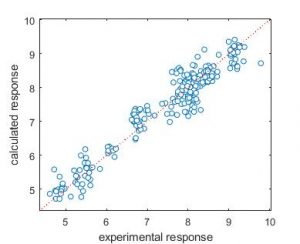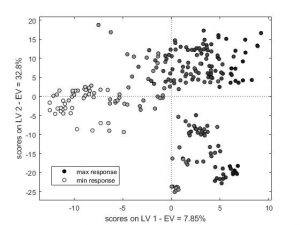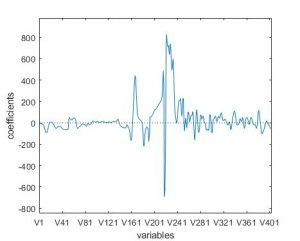# Regression toolbox for MATLAB now available!The Regression toolbox (for MATLAB) is a collection of MATLAB modules for calculating regression multivariate models: Ordinary Least Squares (OLS), Partial Least Squares (PLS), Principal Component Regression (PCR), Ridge regression, local regression based on K Nearest Neighbours (KNN) and Binned Nearest Neighbours (BNN) approaches, and variable selection approaches (All Subset Models, Forward selection, Genetic Algorithms and Reshaped Sequential Replacement). The toolbox is freely available and can be downloaded here